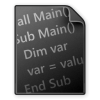Simple Smart Graph Plotter
(by jingzhi)## Resource File

174
Support Email

I needed to plot a line graph (similar to excel) in Rhino with labels and axis. As simple of a task as it is, tools online require so many inputs / parameters. Hence, this script aims to plot a minimalistic graph with only the essentials.

Screenshot captures what I am trying to do.

The inputs are:

1. A set of points - X and Y coordinates of points will be extracted to plot on graph.
2. 1 rectangle - to define the size of plot. Text and axes sizes adjusts prametrically.

Enjoy plotting!

• Category:
Resource Type
: